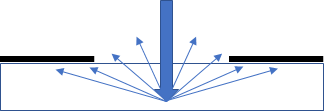## Yule-Nielsen

### Quick Start

If your printed dots were exactly the size you expected, you would be shocked to find that the measured print density value is higher than expected. This is because (on scattering substrates) the white areas between the dots do not return all the light - some gets trapped under your dots. The effect was analysed by Yule and Nielsen and involves a parameter, n, which is 1 on a non-scattering substrate and, in theory, 2 on a scattering one.

In reality you also have real dot gain. The app lets you mix and match Yule-Nielsen and real gain. Specify a "real" 50% dot in terms of size (say it's 70, giving a 20% dot gain), the whole dot gain curve is estimated as if n=1. Then Yule-Nielsen can be added and you get the whole picture. When the combined dot gains give you a 100% dot at a lower printed density, you lose part of your tonal range, shown as % Tone Reduction.

### Yule-Nielsen

Real Dot50
Yule-Nielsen n
Solid Density
%Tone ReductionIf you happen to have printed a perfect 50% dot without any dot gain and proudly measure it with a densitometer, you will be dismayed to find that it has an effective dot area of, say, 60%. This means that more light is being absorbed by the print than just by the dots themselves. Or, to put it another way, some of the light that should have been reflected from the substrate has gone missing. As the graphic shows, that light should have been reflected back from the open areas but via scattering got trapped under the dots

Clearly the effect will be stronger when dots are closer together in prints with higher screen rulings and also in the darker parts of the print

To put this Yule-Nielsen (Y-N) effect into an app it is useful to combine it with the Murray-Davis equation linking printed dot area to densitometer measurements and with a classic dot gain curve such as the GRl equation from Viggiano at the Graphics Research Lab in Rochester Institute of Technology. We can then look at how the "real" and "optical" gains influence the measured print.

GRL is convenient because it gives us an entire, plausible, dot gain curve at each area, A, ΔA from one value - the measured % gain for a printed 50% dot, Δ50.

Δ_A=2Δ_50sqrt(A(1-A))

You can see this curve by setting n=1. GRL also includes a value for where the curve hits 100%, i.e. you print solids instead of half tones. This is discussed below.

If you set Δ50 to 0, i.e. you have an ideal print, you can then explore the Y-N effect by varying n. The Y-N equation is the Murray-Davis equation with 1/n terms included - i.e. if n=1 then the equation is pure M-D. For a solid optical density DS (one of the inputs) and a measured density D, then the real area is:

A=((1-10^(-D/n)))/(1-10^(-"DS"/n))

Play with n=1 and vary Δ50, then play with Δ50=0 and vary n. Only then should you try to combine them.

I found the results confusing at first but they started to make sense. With Δ50=0 you see that you get an optical gain (O-gain) as you increase n and you also get a confusing Ideal-Y-N curve. This is how the real dots would have to be printed in order to get an ideal curve. The effect of DS is to make the effects of optical gain worse at higher values of A.

Y-N tells us that prints should vary from n=1 for smooth substrates to n=2 for highly scattering substrates, and that n is larger for finer halftones. Unfortunately, many prints require higher n values to fit the data. The most plausible explanation is contributions from ink scatter and from ragged dot edges.

The GRL equation identifies Amax above which everything is solid. This has an impact on the tonal range you can print after correction. Suppose Amax=80%. Sure, you can print from 0-100% measured values by printing dots from 0-80%. What you lose is tonal resolution. If your imagesetter gives meaningful steps of 5% then you have gone from a range of 20 different shades to 16. Whether this matters to you depends on how demanding your customers are about fine tonal ranges. The Tone Reduction box is a reminder of what % you have lost.

The point of this app is not to give you an infallible tool for real prints. Instead it is to give you a feel for:

1. How real dot gain and optical gain combine
2. How any real dot gain will get worse at finer halftones
3. How you can clip the tonal range more that you would imagine with just real dot gain
4. Why good control of ink scatter and dot edge shape (which affect n) is important to stay in good control